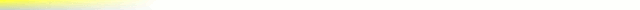# [mkgmap-dev] Curvy routing support: new function?

From Manfred Brenneisen Manfred.Brenneisen at gmx.net on Tue Jul 1 21:02:42 BST 2014

```Hi all,

Garmin offers "curvy roads" preferences for their zümo 390 and 590 devices.
I'm thinking about creating motorcycle maps for old 200 series.
Might it be useful to integrate a curviness() function so that mkgmap can optimize for curvy roads too? It might also help do determine a better default speed for curvy roads for use with car routing.

The curviness() value might be the overall absolute turning angle (in degrees) of a road segment divided by the segment's length.

Cheers
Manfred

Pseudo code might look like this:

function curviness()
{
oldpoint=points(0)
foreach (point of a way segment as newpoint)
{
d_x=(newpoint.x-oldpoint.x)/360*40000000*COS(newpoint.x*PI()/180) //in meters
d_y=(newpoint.y-oldpoint.y)/360*40000000 //in meters
length+=sqrt(d_x**2+d_y**2)
angle=arctan(d_y/d_x)
d_angle=abs(angle-old_angle)
sgn_x=sgn(d_x)
sgn_y=sgn(d_y)
if ((sgn_x*sgn_y*old_sgn_x*old_sgn_y)=-1 && (sgn_x!=old_sgn_x))  d_angle=pi-d_angle  // 180 degrees correction, otherwise north-south roads show strange results
if (point>1) sum_angle+=d_angle
old_angle=angle
oldpoint=newpoint
old_sgn_x=sgn_x
old_sgn_y=sgn_y
}
return sum_angle/length
}

// perhaps simple multiplication is faster than 4 times sgn() functions?
```